# Telangana SSC Board (TS SSC) Question Paper 1 for Class 10th Maths 2017 In PDF

## Telangana 10th Annual Exam Question Papers 2017 Maths Paper 1 with Solutions – Free Download

Telangana SSC Class 10 Maths 2017 Question Paper 1 with solutions are provided by the expert faculty at BYJU’S. Students can get the answers to all questions that have appeared in the TS SSC Question Paper 2017 Maths Paper 1 at BYJU’S. Besides, a number of previous year question papers of Telangana SSC (Class 10) Maths along with the solutions are available to help the students in understanding the exam pattern. All the Telangana 10th Annual Exam Question Papers 2017 Maths Paper 1 solutions have detailed explanations with the necessary images and graphs, so that students can understand them easily. Students are also allowed to get the practise questions of Class 10 Maths along with the important concepts of Maths at BYJU’S.

### Telangana Board SSC Class 10 Maths 2017 Question Paper 1 with Solutions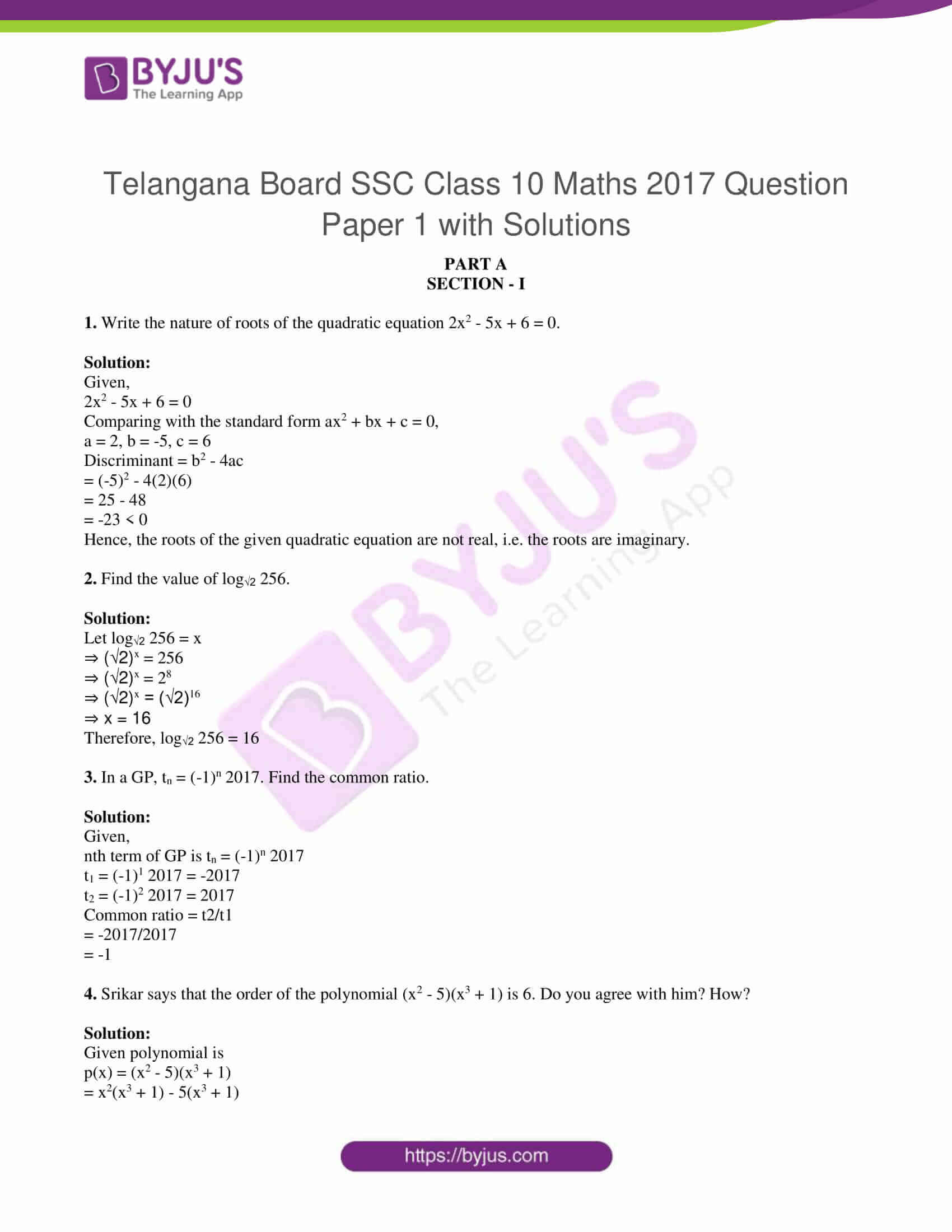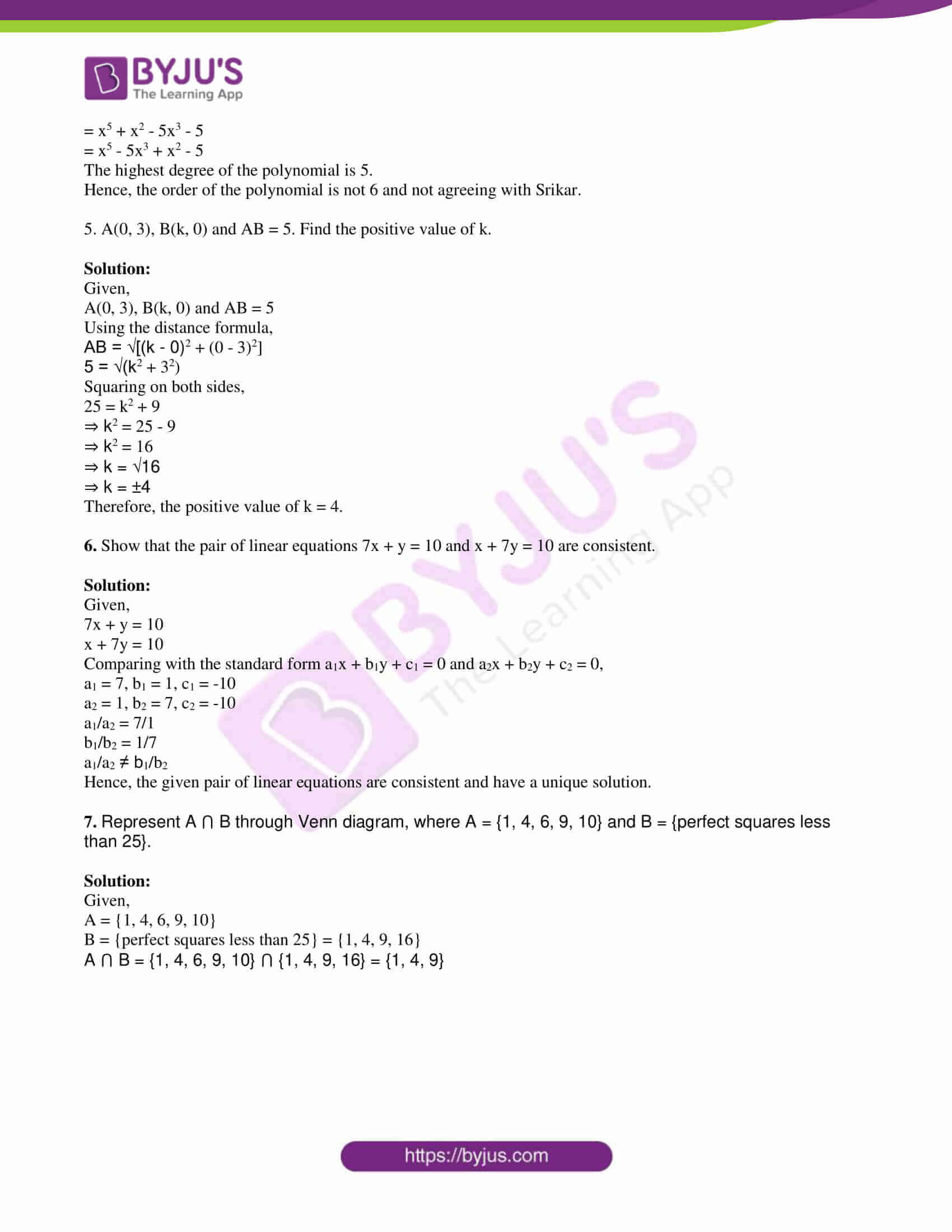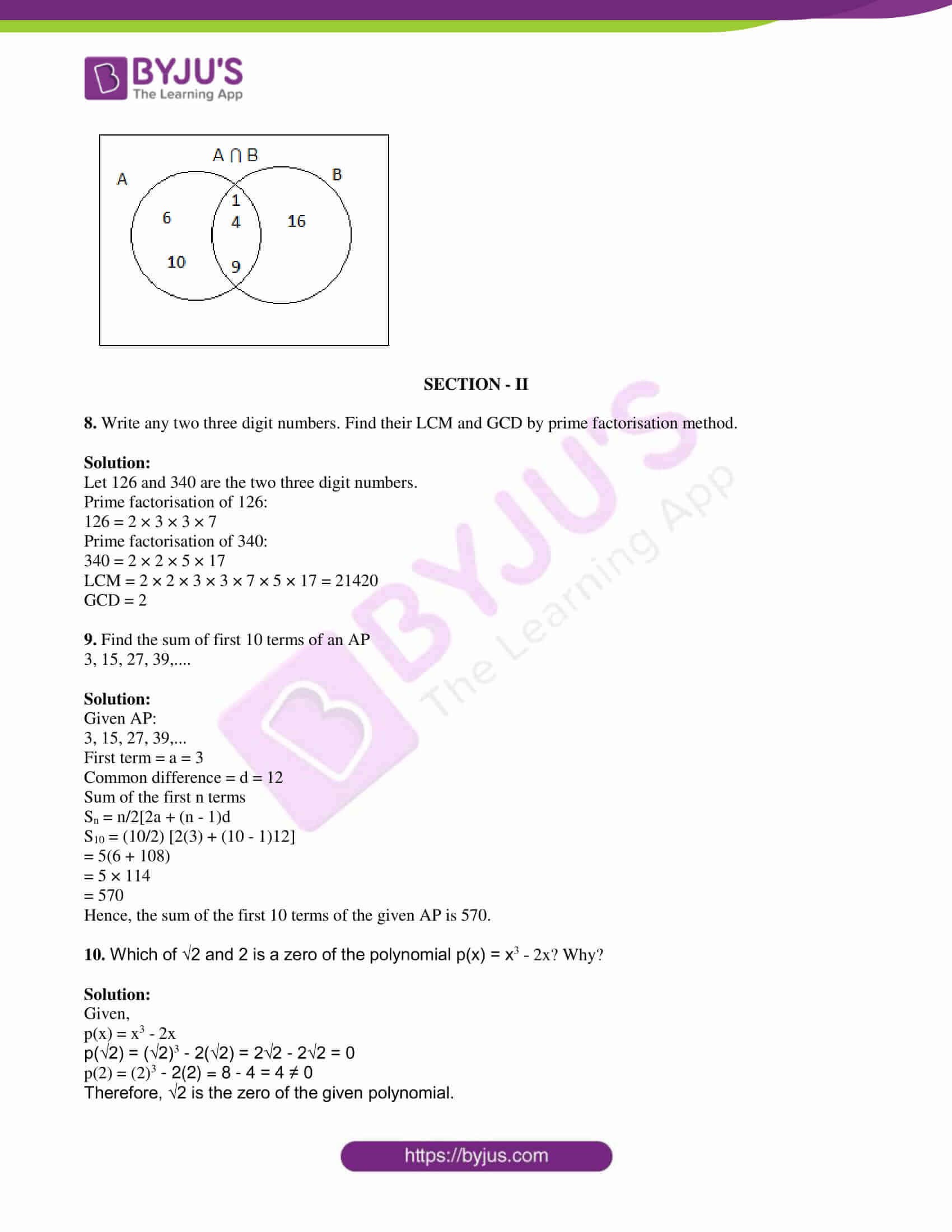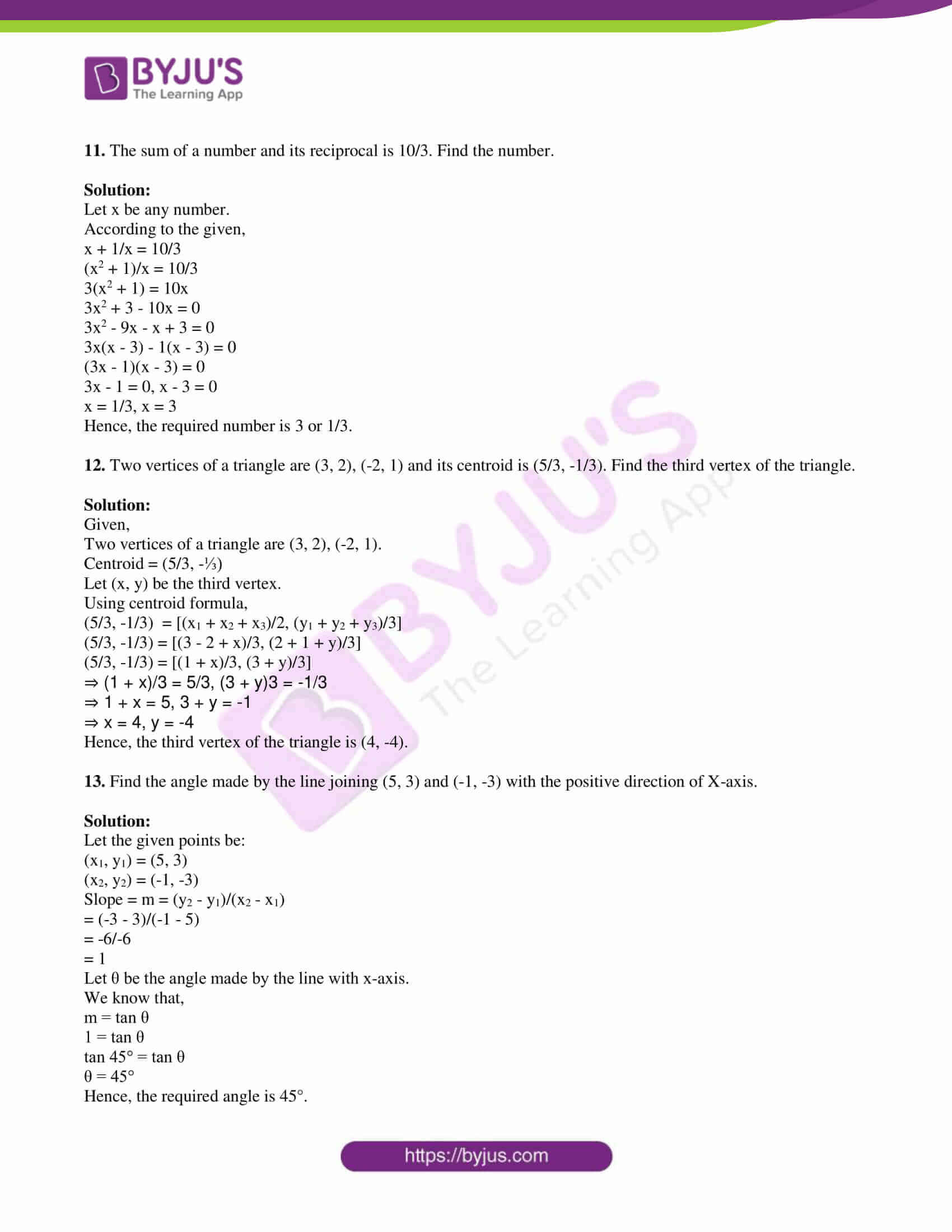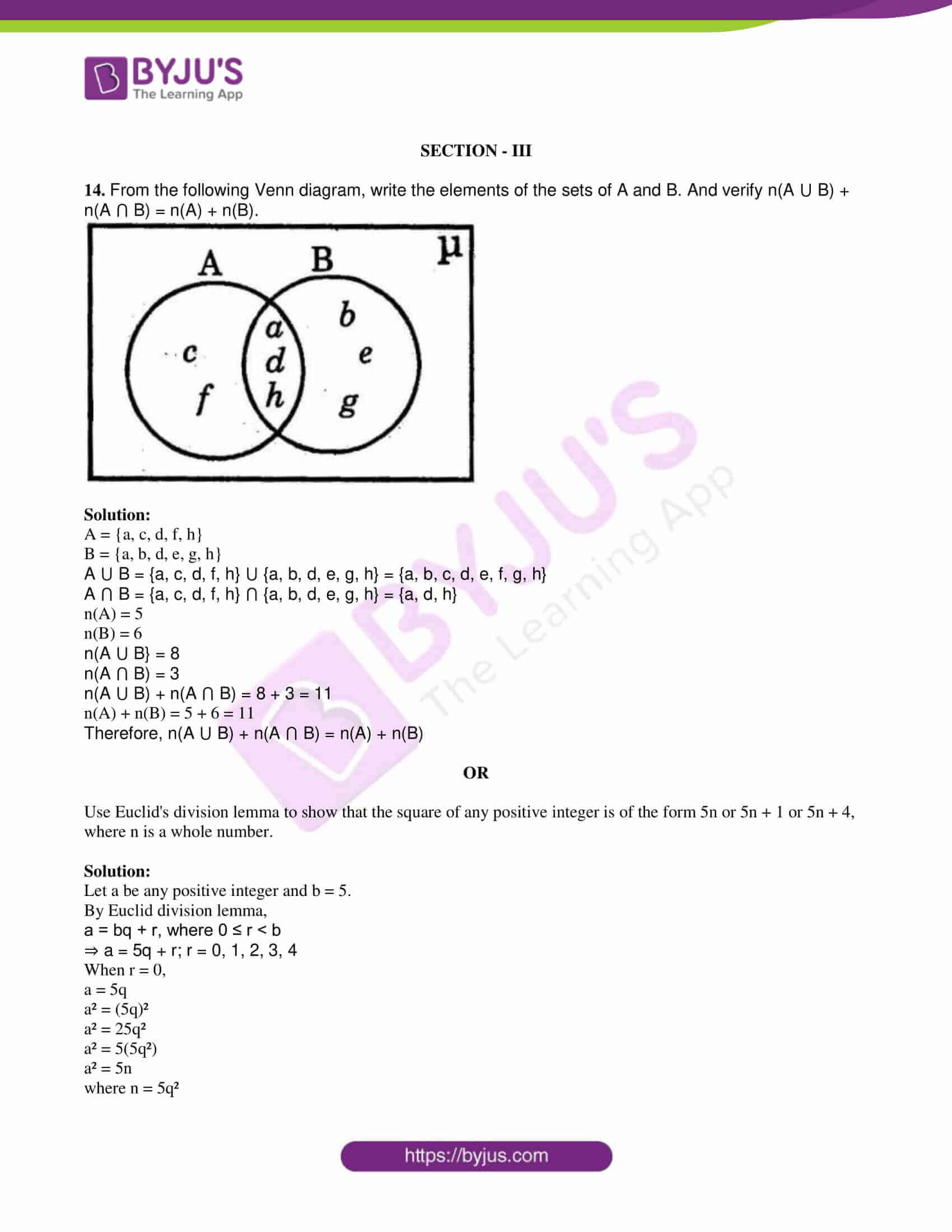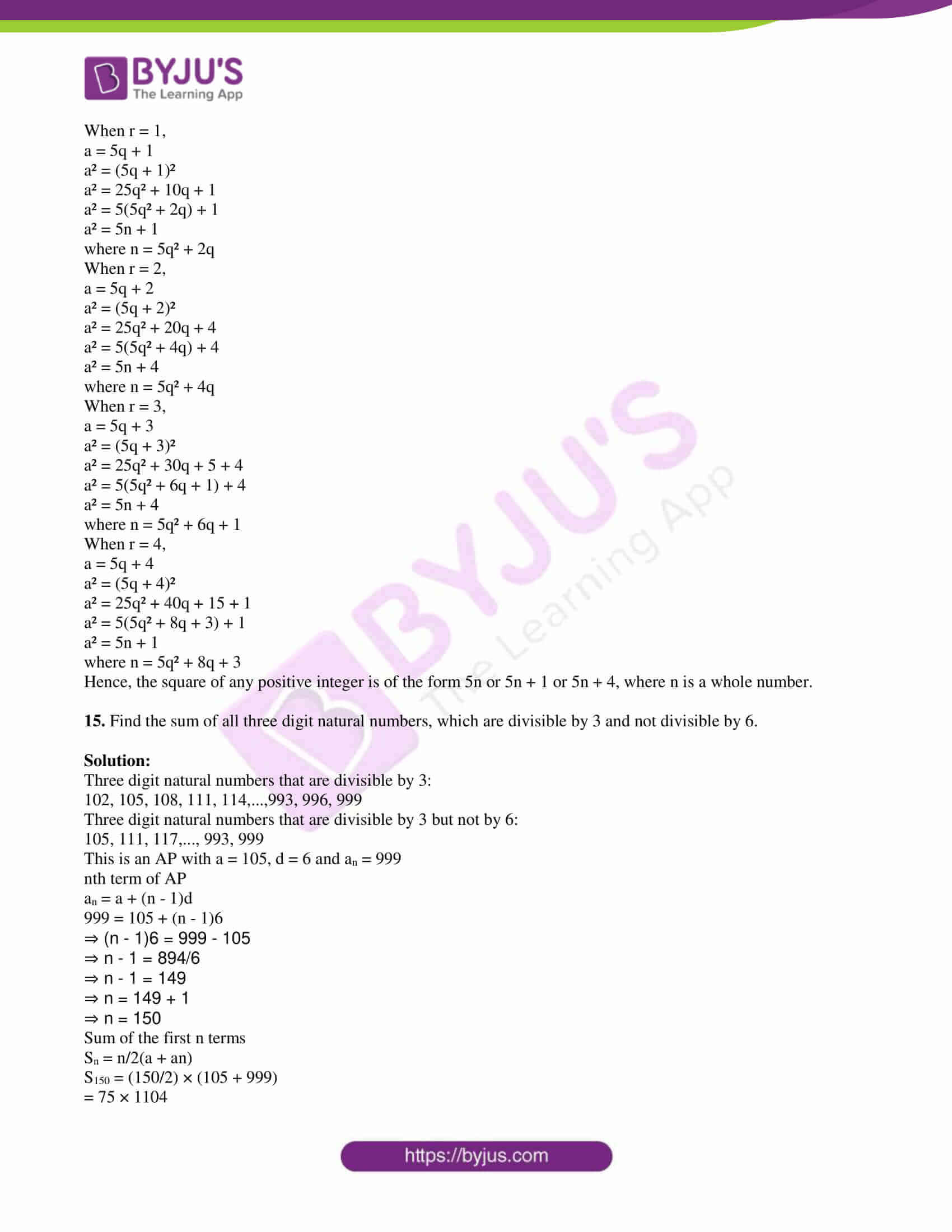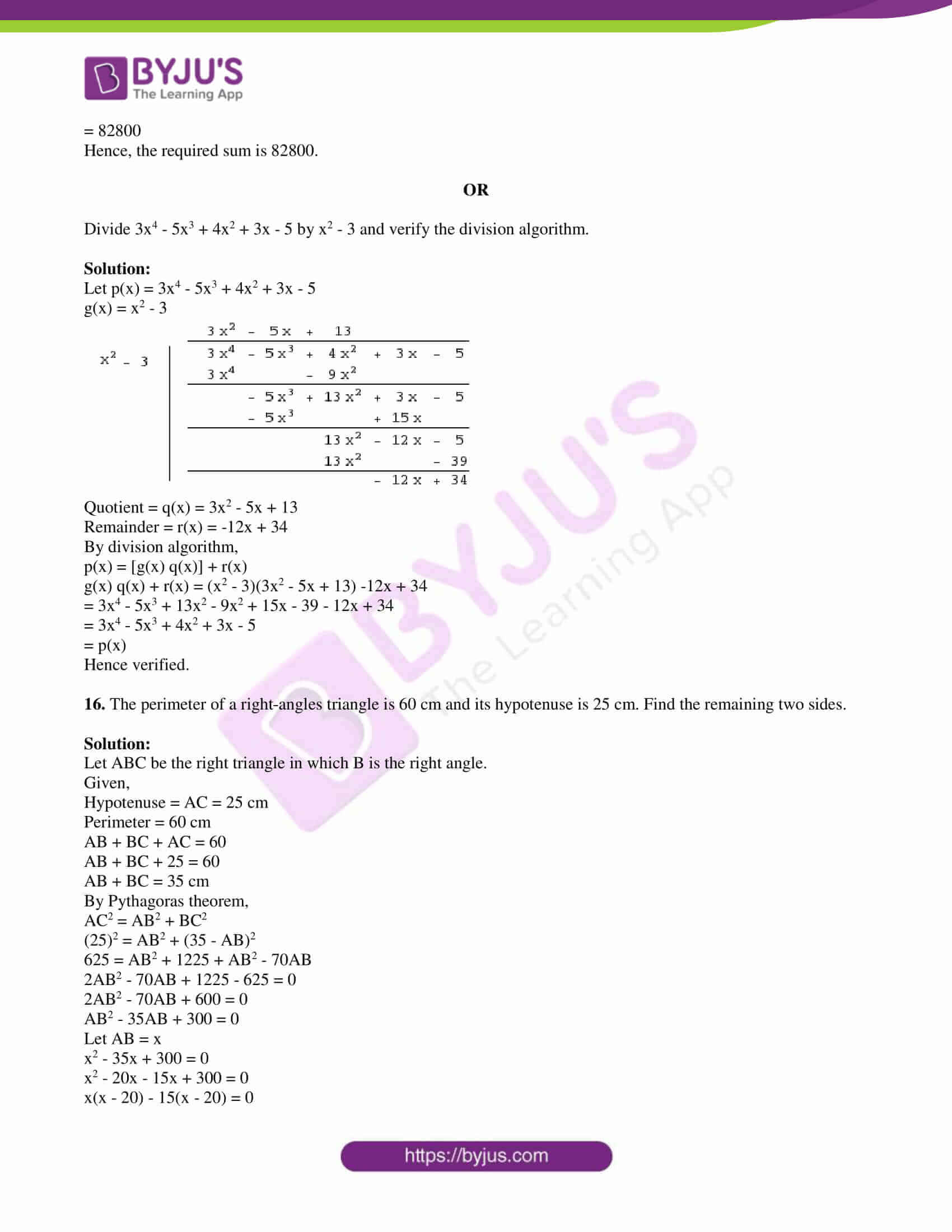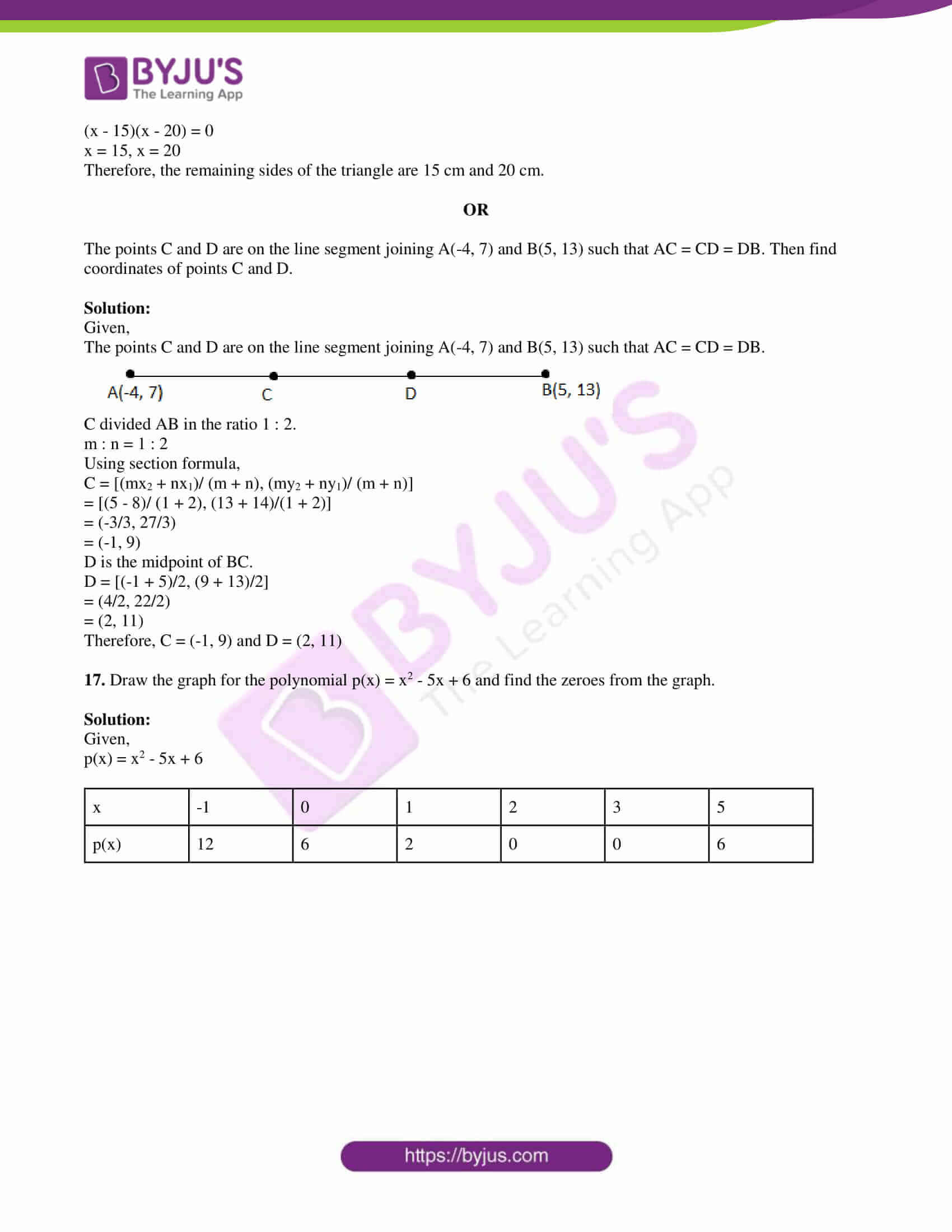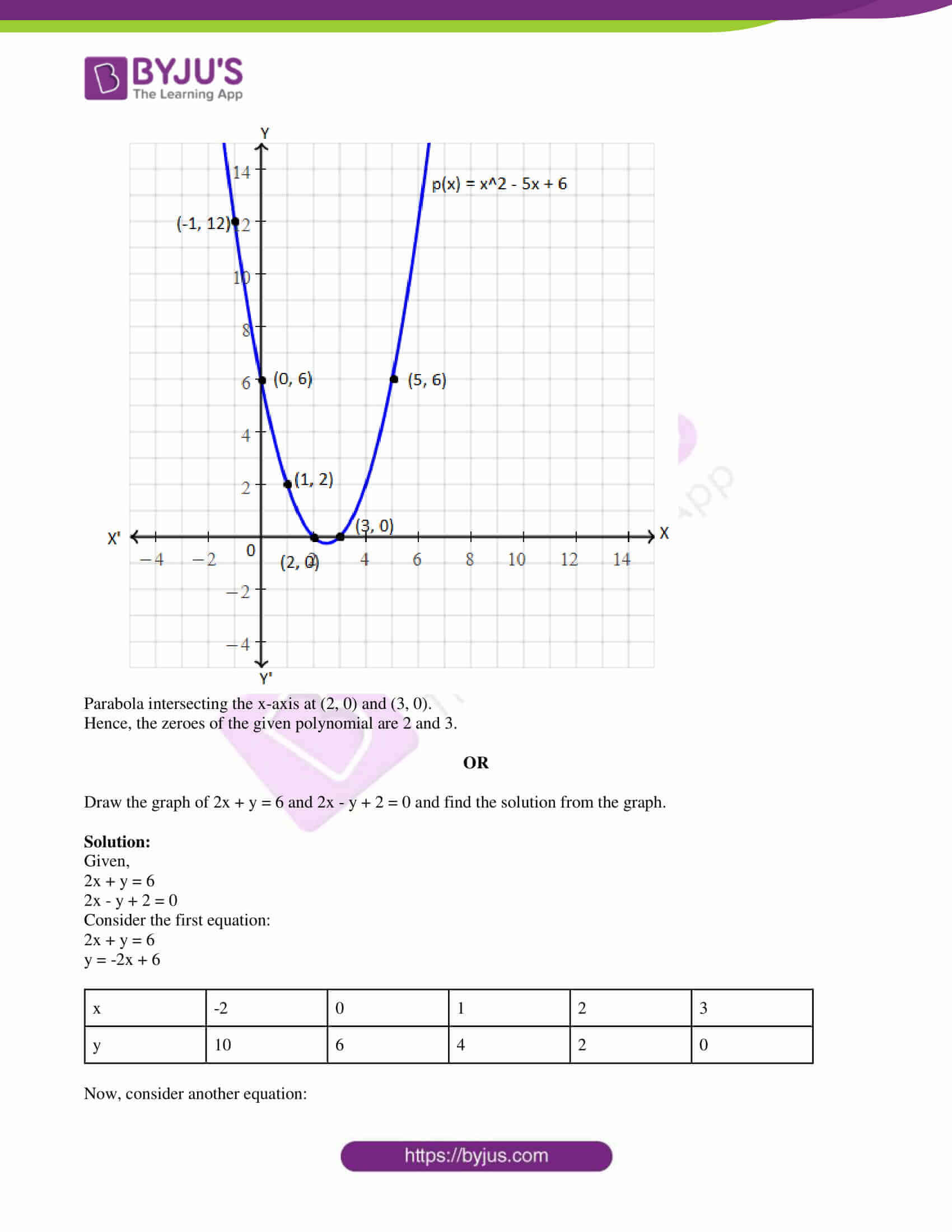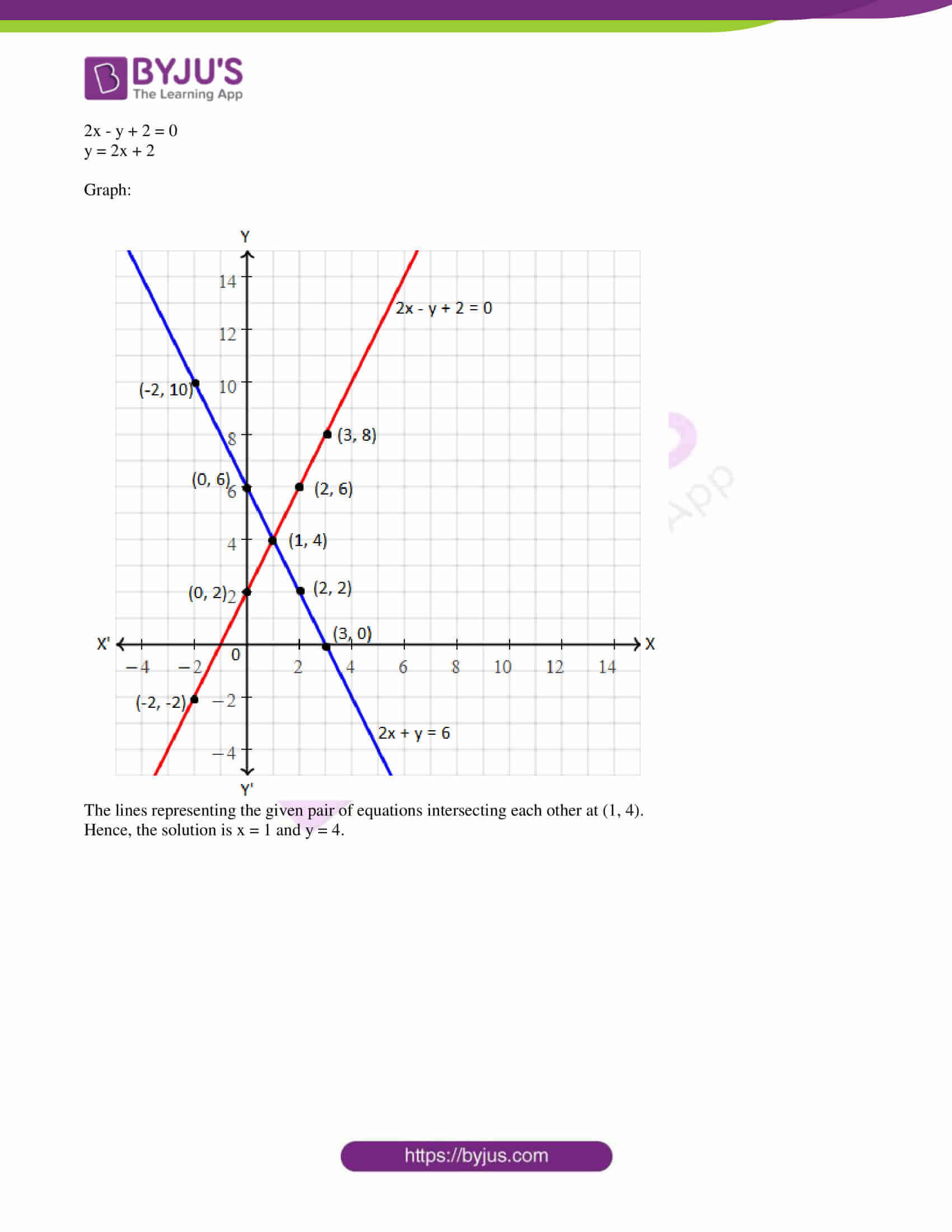PART A

SECTION – I

1. Write the nature of roots of the quadratic equation 2x2 – 5x + 6 = 0.

Solution:

Given,

2x2 – 5x + 6 = 0

Comparing with the standard form ax2 + bx + c = 0,

a = 2, b = -5, c = 6

Discriminant = b2 – 4ac

= (-5)2 – 4(2)(6)

= 25 – 48

= -23 < 0

Hence, the roots of the given quadratic equation are not real, i.e. the roots are imaginary.

2. Find the value of log√2 256.

Solution:

Let log√2 256 = x

⇒ (√2)x = 256

⇒ (√2)x = 28

⇒ (√2)x = (√2)16

⇒ x = 16

Therefore, log√2 256 = 16

3. In a GP, tn = (-1)n 2017. Find the common ratio.

Solution:

Given,

nth term of GP is tn = (-1)n 2017

t1 = (-1)1 2017 = -2017

t2 = (-1)2 2017 = 2017

Common ratio = t2/t1

= -2017/2017

= -1

4. Srikar says that the order of the polynomial (x2 – 5)(x3 + 1) is 6. Do you agree with him? How?

Solution:

Given polynomial is

p(x) = (x2 – 5)(x3 + 1)

= x2(x3 + 1) – 5(x3 + 1)

= x5 + x2 – 5x3 – 5

= x5 – 5x3 + x2 – 5

The highest degree of the polynomial is 5.

Hence, the order of the polynomial is not 6 and not agreeing with Srikar.

5. A(0, 3), B(k, 0) and AB = 5. Find the positive value of k.

Solution:

Given,

A(0, 3), B(k, 0) and AB = 5

Using the distance formula,

AB = √[(k – 0)2 + (0 – 3)2]

5 = √(k2 + 32)

Squaring on both sides,

25 = k2 + 9

⇒ k2 = 25 – 9

⇒ k2 = 16

⇒ k = √16

⇒ k = ±4

Therefore, the positive value of k = 4.

6. Show that the pair of linear equations 7x + y = 10 and x + 7y = 10 are consistent.

Solution:

Given,

7x + y = 10

x + 7y = 10

Comparing with the standard form a1x + b1y + c1 = 0 and a2x + b2y + c2 = 0,

a1 = 7, b1 = 1, c1 = -10

a2 = 1, b2 = 7, c2 = -10

a1/a2 = 7/1

b1/b2 = 1/7

a1/a2 ≠ b1/b2

Hence, the given pair of linear equations are consistent and have a unique solution.

7. Represent A ⋂ B through Venn diagram, where A = {1, 4, 6, 9, 10} and B = {perfect squares less than 25}.

Solution:

Given,

A = {1, 4, 6, 9, 10}

B = {perfect squares less than 25} = {1, 4, 9, 16}

A ⋂ B = {1, 4, 6, 9, 10} ⋂ {1, 4, 9, 16} = {1, 4, 9}SECTION – II

8. Write any two three digit numbers. Find their LCM and GCD by prime factorisation method.

Solution:

Let 126 and 340 are the two three digit numbers.

Prime factorisation of 126:

126 = 2 × 3 × 3 × 7

Prime factorisation of 340:

340 = 2 × 2 × 5 × 17

LCM = 2 × 2 × 3 × 3 × 7 × 5 × 17 = 21420

GCD = 2

9. Find the sum of first 10 terms of an AP

3, 15, 27, 39,….

Solution:

Given AP:

3, 15, 27, 39,…

First term = a = 3

Common difference = d = 12

Sum of the first n terms

Sn = n/2[2a + (n – 1)d

S10 = (10/2) [2(3) + (10 – 1)12]

= 5(6 + 108)

= 5 × 114

= 570

Hence, the sum of the first 10 terms of the given AP is 570.

10. Which of √2 and 2 is a zero of the polynomial p(x) = x3 – 2x? Why?

Solution:

Given,

p(x) = x3 – 2x

p(√2) = (√2)3 – 2(√2) = 2√2 – 2√2 = 0

p(2) = (2)3 – 2(2) = 8 – 4 = 4 ≠ 0

Therefore, √2 is the zero of the given polynomial.

11. The sum of a number and its reciprocal is 10/3. Find the number.

Solution:

Let x be any number.

According to the given,

x + 1/x = 10/3

(x2 + 1)/x = 10/3

3(x2 + 1) = 10x

3x2 + 3 – 10x = 0

3x2 – 9x – x + 3 = 0

3x(x – 3) – 1(x – 3) = 0

(3x – 1)(x – 3) = 0

3x – 1 = 0, x – 3 = 0

x = 1/3, x = 3

Hence, the required number is 3 or 1/3.

12. Two vertices of a triangle are (3, 2), (-2, 1) and its centroid is (5/3, -1/3). Find the third vertex of the triangle.

Solution:

Given,

Two vertices of a triangle are (3, 2), (-2, 1).

Centroid = (5/3, -⅓)

Let (x, y) be the third vertex.

Using centroid formula,

(5/3, -1/3) = [(x1 + x2 + x3)/2, (y1 + y2 + y3)/3]

(5/3, -1/3) = [(3 – 2 + x)/3, (2 + 1 + y)/3]

(5/3, -1/3) = [(1 + x)/3, (3 + y)/3]

⇒ (1 + x)/3 = 5/3, (3 + y)3 = -1/3

⇒ 1 + x = 5, 3 + y = -1

⇒ x = 4, y = -4

Hence, the third vertex of the triangle is (4, -4).

13. Find the angle made by the line joining (5, 3) and (-1, -3) with the positive direction of X-axis.

Solution:

Let the given points be:

(x1, y1) = (5, 3)

(x2, y2) = (-1, -3)

Slope = m = (y2 – y1)/(x2 – x1)

= (-3 – 3)/(-1 – 5)

= -6/-6

= 1

Let θ be the angle made by the line with x-axis.

We know that,

m = tan θ

1 = tan θ

tan 45° = tan θ

θ = 45°

Hence, the required angle is 45°.

SECTION – III

14. From the following Venn diagram, write the elements of the sets of A and B. And verify n(A ⋃ B) + n(A ⋂ B) = n(A) + n(B).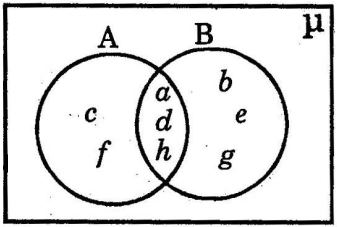Solution:

A = {a, c, d, f, h}

B = {a, b, d, e, g, h}

A ⋃ B = {a, c, d, f, h} ⋃ {a, b, d, e, g, h} = {a, b, c, d, e, f, g, h}

A ⋂ B = {a, c, d, f, h} ⋂ {a, b, d, e, g, h} = {a, d, h}

n(A) = 5

n(B) = 6

n(A ⋃ B} = 8

n(A ⋂ B) = 3

n(A ⋃ B) + n(A ⋂ B) = 8 + 3 = 11

n(A) + n(B) = 5 + 6 = 11

Therefore, n(A ⋃ B) + n(A ⋂ B) = n(A) + n(B)

OR

Use Euclid’s division lemma to show that the square of any positive integer is of the form 5n or 5n + 1 or 5n + 4, where n is a whole number.

Solution:

Let a be any positive integer and b = 5.

By Euclid division lemma,

a = bq + r, where 0 ≤ r < b

⇒ a = 5q + r; r = 0, 1, 2, 3, 4

When r = 0,

a = 5q

a² = (5q)²

a² = 25q²

a² = 5(5q²)

a² = 5n

where n = 5q²

When r = 1,

a = 5q + 1

a² = (5q + 1)²

a² = 25q² + 10q + 1

a² = 5(5q² + 2q) + 1

a² = 5n + 1

where n = 5q² + 2q

When r = 2,

a = 5q + 2

a² = (5q + 2)²

a² = 25q² + 20q + 4

a² = 5(5q² + 4q) + 4

a² = 5n + 4

where n = 5q² + 4q

When r = 3,

a = 5q + 3

a² = (5q + 3)²

a² = 25q² + 30q + 5 + 4

a² = 5(5q² + 6q + 1) + 4

a² = 5n + 4

where n = 5q² + 6q + 1

When r = 4,

a = 5q + 4

a² = (5q + 4)²

a² = 25q² + 40q + 15 + 1

a² = 5(5q² + 8q + 3) + 1

a² = 5n + 1

where n = 5q² + 8q + 3

Hence, the square of any positive integer is of the form 5n or 5n + 1 or 5n + 4, where n is a whole number.

15. Find the sum of all three digit natural numbers, which are divisible by 3 and not divisible by 6.

Solution:

Three digit natural numbers that are divisible by 3:

102, 105, 108, 111, 114,…,993, 996, 999

Three digit natural numbers that are divisible by 3 but not by 6:

105, 111, 117,…, 993, 999

This is an AP with a = 105, d = 6 and an = 999

nth term of AP

an = a + (n – 1)d

999 = 105 + (n – 1)6

⇒ (n – 1)6 = 999 – 105

⇒ n – 1 = 894/6

⇒ n – 1 = 149

⇒ n = 149 + 1

⇒ n = 150

Sum of the first n terms

Sn = n/2(a + an)

S150 = (150/2) × (105 + 999)

= 75 × 1104

= 82800

Hence, the required sum is 82800.

OR

Divide 3x4 – 5x3 + 4x2 + 3x – 5 by x2 – 3 and verify the division algorithm.

Solution:

Let p(x) = 3x4 – 5x3 + 4x2 + 3x – 5

g(x) = x2 – 3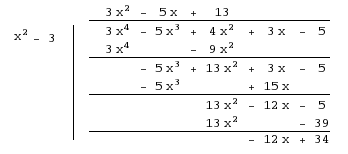Quotient = q(x) = 3x2 – 5x + 13

Remainder = r(x) = -12x + 34

By division algorithm,

p(x) = [g(x) q(x)] + r(x)

g(x) q(x) + r(x) = (x2 – 3)(3x2 – 5x + 13) -12x + 34

= 3x4 – 5x3 + 13x2 – 9x2 + 15x – 39 – 12x + 34

= 3x4 – 5x3 + 4x2 + 3x – 5

= p(x)

Hence verified.

16. The perimeter of a right-angles triangle is 60 cm and its hypotenuse is 25 cm. Find the remaining two sides.

Solution:

Let ABC be the right triangle in which B is the right angle.

Given,

Hypotenuse = AC = 25 cm

Perimeter = 60 cm

AB + BC + AC = 60

AB + BC + 25 = 60

AB + BC = 35 cm

By Pythagoras theorem,

AC2 = AB2 + BC2

(25)2 = AB2 + (35 – AB)2

625 = AB2 + 1225 + AB2 – 70AB

2AB2 – 70AB + 1225 – 625 = 0

2AB2 – 70AB + 600 = 0

AB2 – 35AB + 300 = 0

Let AB = x

x2 – 35x + 300 = 0

x2 – 20x – 15x + 300 = 0

x(x – 20) – 15(x – 20) = 0

(x – 15)(x – 20) = 0

x = 15, x = 20

Therefore, the remaining sides of the triangle are 15 cm and 20 cm.

OR

The points C and D are on the line segment joining A(-4, 7) and B(5, 13) such that AC = CD = DB. Then find coordinates of points C and D.

Solution:

Given,

The points C and D are on the line segment joining A(-4, 7) and B(5, 13) such that AC = CD = DB.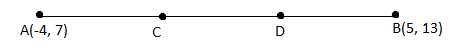C divided AB in the ratio 1 : 2.

m : n = 1 : 2

Using section formula,

C = [(mx2 + nx1)/ (m + n), (my2 + ny1)/ (m + n)]

= [(5 – 8)/ (1 + 2), (13 + 14)/(1 + 2)]

= (-3/3, 27/3)

= (-1, 9)

D is the midpoint of BC.

D = [(-1 + 5)/2, (9 + 13)/2]

= (4/2, 22/2)

= (2, 11)

Therefore, C = (-1, 9) and D = (2, 11)

17. Draw the graph for the polynomial p(x) = x2 – 5x + 6 and find the zeroes from the graph.

Solution:

Given,

p(x) = x2 – 5x + 6

 x -1 0 1 2 3 5 p(x) 12 6 2 0 0 6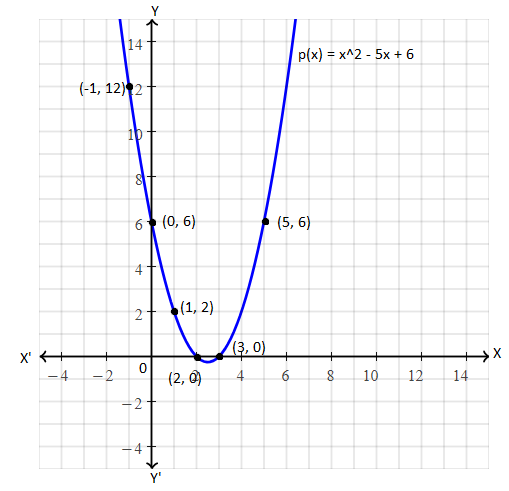Parabola intersecting the x-axis at (2, 0) and (3, 0).

Hence, the zeroes of the given polynomial are 2 and 3.

OR

Draw the graph of 2x + y = 6 and 2x – y + 2 = 0 and find the solution from the graph.

Solution:

Given,

2x + y = 6

2x – y + 2 = 0

Consider the first equation:

2x + y = 6

y = -2x + 6

 x -2 0 1 2 3 y 10 6 4 2 0

Now, consider another equation:

2x – y + 2 = 0

y = 2x + 2

Graph: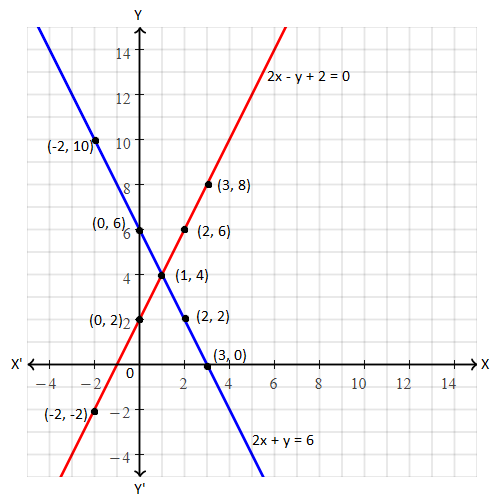The lines representing the given pair of equations intersecting each other at (1, 4).

Hence, the solution is x = 1 and y = 4.